# Electricity Basics

## Electricity Basics

When beginning to explore the world of electricity and electronics, it is vital to start by understanding the basics of voltage, current, and resistance. These are the three basic building blocks required to manipulate and utilize electricity. At first, these concepts can be difficult to understand because we cannot “see” them. One cannot see with the naked eye the energy flowing through a wire or the voltage of a battery sitting on a table. Even the lightning in the sky, while visible, is not truly the energy exchange happening from the clouds to the earth, but a reaction in the air to the energy passing through it. In order to detect this energy transfer, we must use measurement tools such as multimeters, spectrum analyzers, and oscilloscopes to visualize what is happening with the charge in a system. Fear not, however, this tutorial will give you the basic understanding of voltage, current, and resistance and how the three relate to each other.

There are two types of electrical  signals , those being Alternating current (AC), and Direct current (DC).

With Alternating current, the direction electricity flows throughout the circuit is constantly reversing. You may even say that it is alternating direction. The rate of reversal is measured in Hertz, which is the number of reversals per second. So, when they say that the US power supply is 60 Hz, what they mean is that it is reversing 120 times per second (twice per cycle).

With Direct Current, electricity flows in one direction between power and ground. In this arrangement there is always a positive source of voltage and ground (0V) source of voltage. You can test this by reading a battery with a multimeter. For great instructions on how to do this, check out Ladyada’s multimeter page (you will want to measure voltage in particular).

Speaking of voltage, electricity is typically defined as having a voltage and a current rating. Voltage is obviously rated in Volts and current is rated in Amps. For instance, a brand new 9V battery would have a voltage of 9V and a current of around 500mA (500 milliamps).

Electricity can also be defined in terms of resistance and watts. We will talk a little bit about resistance in the next step, but I am not going to be going over Watts in depth. As you delve deeper into electronics you will encounter components with Watt ratings. It is important to never exceed the Wattage rating of a component, but fortunately that Wattage of your DC power supply can easily be calculated by multiplying the voltage and current of your power source.

Most basic electronic circuits use DC electricity. As such, all further discussion of electricity will revolve around DC electricity.

## Electrical Charge

Electricity is the movement of electrons. Electrons create charge, which we can harness to do work. Your lightbulb, your stereo, your phone, etc., are all harnessing the movement of the electrons in order to do work. They all operate using the same basic power source: the movement of electrons.

The three basic principles for this tutorial can be explained using electrons, or more specifically, the charge they create:

• Voltage is the difference in charge between two points.
• Current is the rate at which charge is flowing.
• Resistance is a material’s tendency to resist the flow of charge (current).

So, when we talk about these values, we’re really describing the movement of charge, and thus, the behavior of electrons. A circuit is a closed loop that allows charge to move from one place to another. Components in the circuit allow us to control this charge and use it to do work.

Georg Ohm was a Bavarian scientist who studied electricity. Ohm starts by describing a unit of resistance that is defined by current and voltage. So, let’s start with voltage and go from there.

## Voltage

We define voltage as the amount of potential energy between two points on a circuit. One point has more charge than another. This difference in charge between the two points is called voltage. It is measured in volts, which, technically, is the potential energy difference between two points that will impart one joule of energy per coulomb of charge that passes through it (don’t panic if this makes no sense, all will be explained). The unit “volt” is named after the Italian physicist Alessandro Volta who invented what is considered the first chemical battery. Voltage is represented in equations and schematics by the letter “V”.

When describing voltage, current, and resistance, a common analogy is a water tank. In this analogy, charge is represented by the water amount, voltage is represented by the water pressure, and current is represented by the water flow. So for this analogy, remember:

• Water = Charge
• Pressure = Voltage
• Flow = Current

Consider a water tank at a certain height above the ground. At the bottom of this tank there is a hose.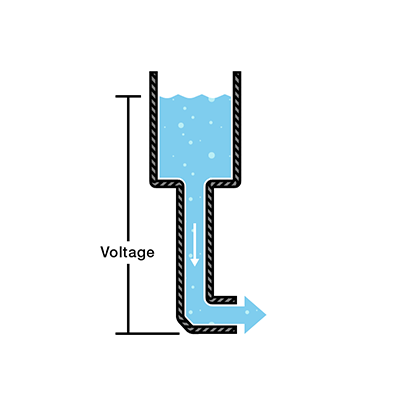The pressure at the end of the hose can represent voltage. The water in the tank represents charge. The more water in the tank, the higher the charge, the more pressure is measured at the end of the hose.

We can think of this tank as a battery, a place where we store a certain amount of energy and then release it. If we drain our tank a certain amount, the pressure created at the end of the hose goes down. We can think of this as decreasing voltage, like when a flashlight gets dimmer as the batteries run down. There is also a decrease in the amount of water that will flow through the hose. Less pressure means less water is flowing, which brings us to current.

## Current

We can think of the amount of water flowing through the hose from the tank as current. The higher the pressure, the higher the flow, and vice-versa. With water, we would measure the volume of the water flowing through the hose over a certain period of time. With electricity, we measure the amount of charge flowing through the circuit over a period of time. Current is measured in Amperes (usually just referred to as “Amps”). An ampere is defined as 6.241*1018 electrons (1 Coulomb) per second passing through a point in a circuit. Amps are represented in equations by the letter “I”.

Let’s say now that we have two tanks, each with a hose coming from the bottom. Each tank has the exact same amount of water, but the hose on one tank is narrower than the hose on the other.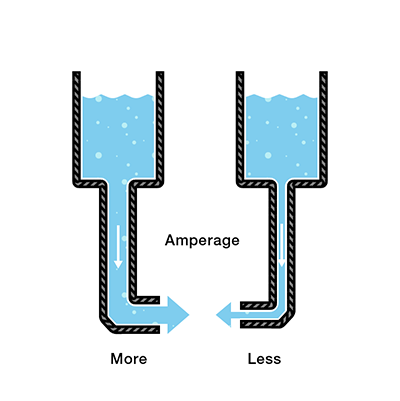We measure the same amount of pressure at the end of either hose, but when the water begins to flow, the flow rate of the water in the tank with the narrower hose will be less than the flow rate of the water in the tank with the wider hose. In electrical terms, the current through the narrower hose is less than the current through the wider hose. If we want the flow to be the same through both hoses, we have to increase the amount of water (charge) in the tank with the narrower hose.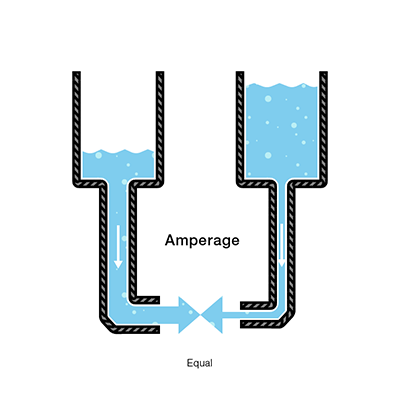This increases the pressure (voltage) at the end of the narrower hose, pushing more water through the tank. This is analogous to an increase in voltage that causes an increase in current.

Now we’re starting to see the relationship between voltage and current. But there is a third factor to be considered here: the width of the hose. In this analogy, the width of the hose is the resistance. This means we need to add another term to our model:

• Water = Charge (measured in Coulombs)
• Pressure = Voltage (measured in Volts)
• Flow = Current (measured in Amperes, or “Amps” for short)
• Hose Width = Resistance

## Resistance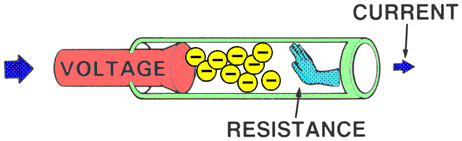Consider again our two water tanks, one with a narrow pipe and one with a wide pipe.It stands to reason that we can’t fit as much volume through a narrow pipe than a wider one at the same pressure. This is resistance. The narrow pipe “resists” the flow of water through it even though the water is at the same pressure as the tank with the wider pipe.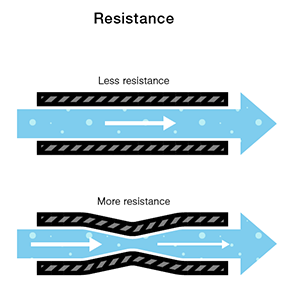In electrical terms, this is represented by two circuits with equal voltages and different resistances. The circuit with the higher resistance will allow less charge to flow, meaning the circuit with higher resistance has less current flowing through it.

This brings us back to Georg Ohm. Ohm defines the unit of resistance of “1 Ohm” as the resistance between two points in a conductor where the application of 1 volt will push 1 ampere, or 6.241×1018electrons. This value is usually represented in schematics with the greek letter “Ω”, which is called omega, and pronounced “ohm”.

## Ohm’s Law

Combining the elements of voltage, current, and resistance, Ohm developed the formula: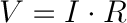Where

• V = Voltage in volts
• I = Current in amps
• R = Resistance in ohms

This is called Ohm’s law. Let’s say, for example, that we have a circuit with the potential of 1 volt, a current of 1 amp, and resistance of 1 ohm. Using Ohm’s Law we can say: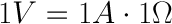Let’s say this represents our tank with a wide hose. The amount of water in the tank is defined as 1 volt and the “narrowness” (resistance to flow) of the hose is defined as 1 ohm. Using Ohms Law, this gives us a flow (current) of 1 amp.

Using this analogy, let’s now look at the tank with the narrow hose. Because the hose is narrower, its resistance to flow is higher. Let’s define this resistance as 2 ohms. The amount of water in the tank is the same as the other tank, so, using Ohm’s Law, our equation for the tank with the narrow hose is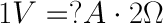But what is the current? Because the resistance is greater, and the voltage is the same, this gives us a current value of 0.5 amps: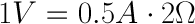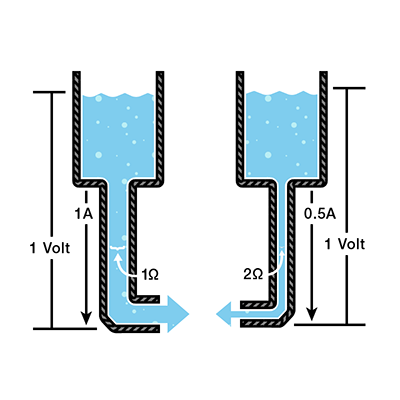So, the current is lower in the tank with higher resistance. Now we can see that if we know two of the values for Ohm’s law, we can solve for the third. Let’s demonstrate this with an experiment.

## An Ohm’s Law Experiment

For this experiment, we want to use a 9 volt battery to power an LED. LEDs are fragile and can only have a certain amount of current flowing through them before they burn out. In the documentation for an LED, there will always be a “current rating”. This is the maximum amount of current that can flow through the particular LED before it burns out.

### Materials Required

In order to perform the experiments listed at the end of the tutorial, you will need:

• A multimeter
• A 9-Volt battery
• A 560-Ohm resistor(or the next closest value)
• An LED

NOTE: LEDs are what’s known as a “non-ohmic” devices. This means that the equation for the current flowing through the LED itself is not as simple as V=IR. The LED introduces something called a “voltage drop” into the circuit, thus changing the amount of current running through it. However, in this experiment we are simply trying to protect the LED from over-current, so we will neglect the current characteristics of the LED and choose the resistor value using Ohm’s Law in order to be sure that the current through the LED is safely under 20mA.

For this example, we have a 9 volt battery and a red LED with a current rating of 20 milliamps, or 0.020 amps. To be safe, we’d rather not drive the LED at its maximum current but rather its suggested current, which is listed on its datasheet as 18mA, or 0.018 amps. If we simply connect the LED directly to the battery, the values for Ohm’s law look like this:therefore: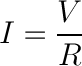and since we have no resistance yet: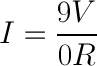Dividing by zero gives us infinite current! Well, not infinite in practice, but as much current as the battery can deliver. Since we do NOT want that much current flowing through our LED, we’re going to need a resistor. Our circuit should look like this: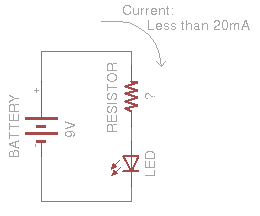We can use Ohm’s Law in the exact same way to determine the reistor value that will give us the desired current value:therefore:plugging in our values: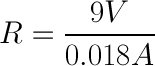solving for resistance:So, we need a resistor value of around 500 ohms to keep the current through the LED under the maximum current rating.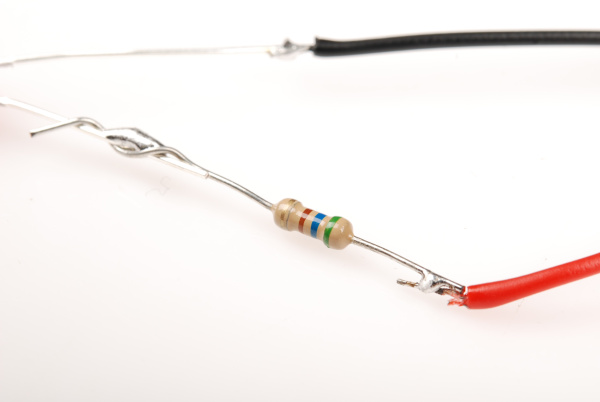500 ohms is not a common value for off-the-shelf resistors, so this device uses a 560 ohm resistor in its place. Here’s what our device looks like all put together.Success! We’ve chosen a resistor value that is high enough to keep the current through the LED below its maximum rating, but low enough that the current is sufficient to keep the LED nice and bright.

This LED/current-limiting resistor example is a common occurrence in hobby electronics. You’ll often need to use Ohm’s Law to change the amount of current flowing through the circuit. Another example of this implementation is seen in the LilyPad LED boards.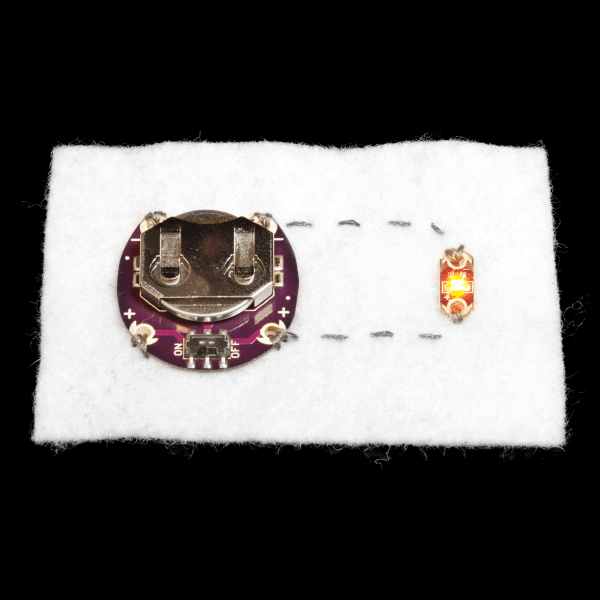With this setup, instead of having to choose the resistor for the LED, the resistor is already on-board with the LED so the current-limiting is accomplished without having to add a resistor by hand.

### Current Limiting Before or After the LED?

To make things a little more complicated, you can place the current limiting resistor on either side of the LED, and it will work just the same!

Many folks learning electronics for the first time struggle with the idea that a current limiting resistor can live on either side of the LED and the circuit will still function as usual.

Imagine a river in a continuous loop, an infinite, circular, flowing river. If we were to place a dam in it, the entire river would stop flowing, not just one side. Now imagine we place a water wheel in the river which slows the flow of the river. It wouldn’t matter where in the circle the water wheel is placed, it will still slow the flow on the entire river.

This is an oversimplification, as the current limiting resistor cannot be placed anywhere in the circuit; it can be placed on either side of the LED to perform its function